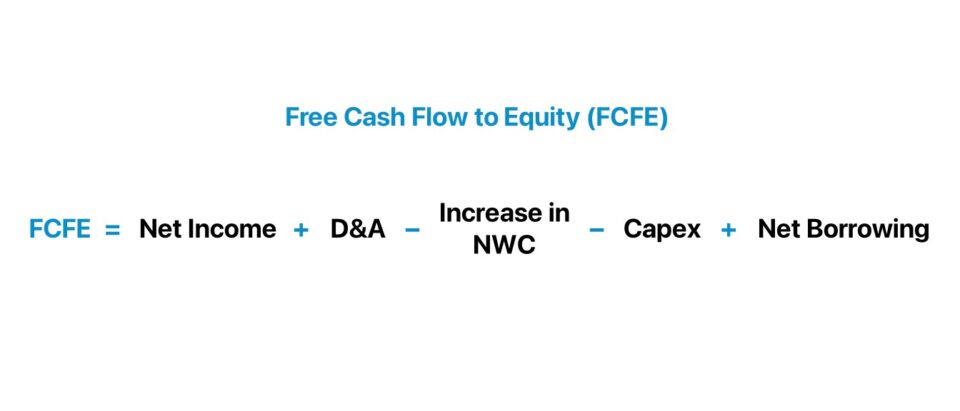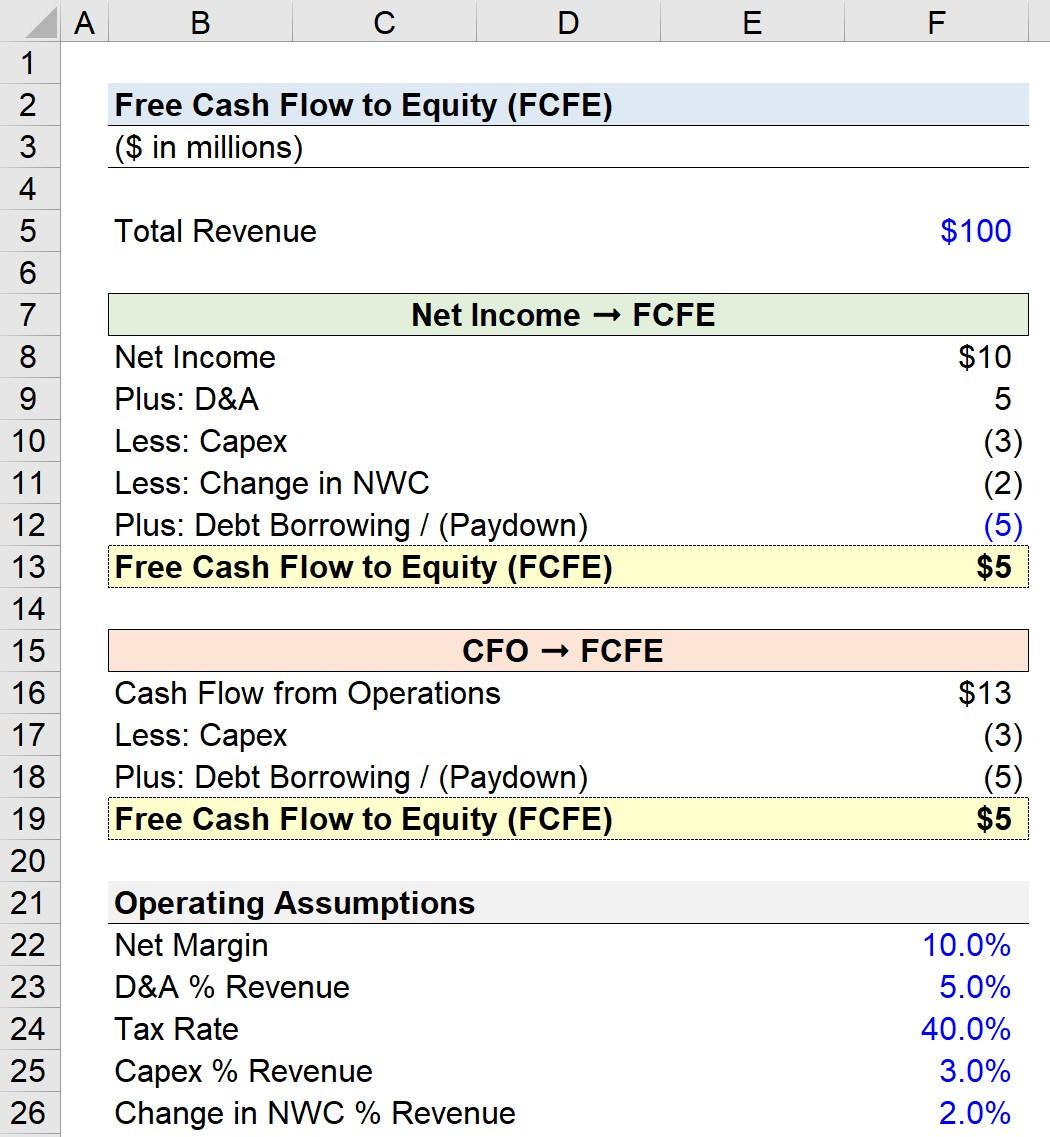Welcome to Wall Street Prep! Use code at checkout for 15% off.# Free Cash Flow to Equity (FCFE)

Guide to Understanding Free Cash Flow to Equity (FCFE)## How to Calculate FCFE?

Since free cash flow to equity (FCFE) represents the cash left over after meeting all financial obligations and re-investment needs to remain operating, such as capital expenditures (Capex) and net working capital, the metric is often used as a proxy for the amount that a company can return to its shareholders via dividends or share buybacks.

The reason for this is that the effects of debt financing have been removed – namely, interest expense, the “tax shield” (i.e., savings from interest being tax-deductible), and principal debt repayments.

Simply put, free cash flow to equity (FCFE) is a “levered” metric, so the value of the cash flows must include the impact of financing obligations.

So, rather than representing the cash available to all capital providers, FCFE is the amount attributable to only equity investors.

For instance, the company could allocate the residual cash flow to fund the following corporate actions:

1. Dividend Issuance → Payout cash dividends directly to preferred and common shareholders
2. Stock Buyback → Buying back shares reduces the shares outstanding, which reduces dilution and can artificially boost the value per share
3. Re-Investments → The company could reinvest the cash into its operations, which in the ideal scenario would increase the share price

The clear pattern is that these actions benefit the equity holders.

Contrast this to interest expense or debt repayments, which solely benefit lenders. That said, the FCFE could be equivalent to the FCFF if there is zero debt in the capital structure.

FCFEs can be projected in a levered discounted cash flow model (DCF) to derive the market value of equity. Furthermore, the correct discount rate to use would be the cost of equity, as the cash flows and discount rate must match up in terms of the represented stakeholders.

However, in practice, the FCFF approach and unlevered DCF are used across most industries. The one notable exception is financial institutions since their main source of revenue is interest income – making it unfeasible to separate the unlevered FCF since the business model itself is oriented around financing (e.g., interest income, interest expense, provision for losses).

## FCFE Formula

The calculation of free cash flow to firm (FCFF) starts with NOPAT, which is a capital-structure-neutral metric.

For FCFE, however, we begin with net income, a metric that has already accounted for the interest expense and tax savings from any debt outstanding.

FCFE = Net Income + D&A Change in NWC Capex + Net Borrowing

Since FCFE is intended to reflect the cash flows that go only to equity holders, there is no need to add back the interest, interest tax shield, or debt repayments. Instead, we simply add back non-cash items, adjust for the change in NWC, and subtract the CapEx amount.

However, another key distinction is the deduction of the net borrowing, which is equal to the debt borrowed net of the repayment.

Net Borrowing = Debt Borrowing Debt Paydown

The reason we include the debt borrowed, as opposed to just the debt paydown, is that the proceeds from the borrowing could be used to distribute dividends or repurchase shares.

In the next approach, the formula for free cash flow to equity (FCFE) starts with cash flow from operations (CFO).

FCFE = Cash from Operations (CFO) Capex + Net Borrowing

Cash from operations (CFO) is calculated by taking net income from the income statement, adding back non-cash charges, and adjusting for the change in NWC, so the remaining steps are to just account for Capex and the net borrowing.

###### Mandatory Amortization vs. Cash Sweep

For the most part, only the mandatory scheduled debt repayments should be included in the calculation of net borrowing.

For example, a cash sweep in an LBO model (i.e., optional repayment of debt) would be excluded because the management could have chosen to use those proceeds instead for other purposes that pertain to equity shareholders.

In comparison, scheduled repayments to lenders are non-discretionary; if they are not paid, the company will default on the debt.

## FCFE Calculator

We’ll now move to a modeling exercise, which you can access by filling out the form below.#### Get the Excel Template!Submitting...

## 1. FCFE Calculation Example (Net Income to FCFE)

Suppose a company’s net income is \$10mm given a 10% net income margin assumption and \$100mm in revenue.

• Total Revenue = \$100 million
• Net Income = \$10 million
• Net Margin = 10%

Next, our D&A assumption of \$5mm is added back since it is a non-cash expense, and then we subtract the \$3mm in Capex and \$2mm increase in NWC.

• D&A = \$5 million
• Capex = \$3 million
• Increase in NWC = \$2 million

That leaves us with \$10mm, but then we must subtract the \$5mm in debt paydown, which leaves us with \$5mm as the FCFE.

• FCFE = \$5 million

## 2. FCFE Calculation Example (CFO to FCFE)

In the 2nd example, we begin with cash from operations (CFO) of \$13mm rather than net income.

CFO is equal to the sum of net income and D&A, subtracted by an increase in NWC, i.e. “cash outflow.”

• Cash from Operations (CFO) = \$10 million + \$5 million – \$2 million = \$13 million

Then, we subtract the \$3mm in Capex and \$5mm in debt paydown to get \$5mm once again.

• FCFE = \$13 million – \$3 million – \$5 million = \$5 million## 3. FCFE Calculation Example (EBITDA to FCFE)

Unlike net income and CFO, EBITDA is capital-structure neutral. Therefore, if we start with EBITDA, we must deduct the impact of debt financing to remove the cash that belongs to lenders.

FCFE = EBITDA Interest Taxes Change in NWC Capex + Net Borrowing

Within the EBITDA metric, the only debt-related component is the interest, which we subtract. Notice that we are just working down the income statement to net income (or the “bottom line”).

That said, the subsequent step is to account for taxes, and there is no need to make additional adjustments to the tax amount as we want to include the interest tax shield.

In the final steps, we’ve moved from EBITDA to net income, so the remaining steps are identical, wherein the change in NWC and Capex are deducted, followed by subtracting the net borrowing for the period to arrive at the FCFE.Step-by-Step Online Course

### Everything You Need To Master Financial Modeling

Enroll in The Premium Package: Learn Financial Statement Modeling, DCF, M&A, LBO and Comps. The same training program used at top investment banks.

Inline Feedbacks
Eugene
June 26, 2022 5:10 am

If you’re doing a DCF valuation, do you still need to add cash on hand and minus total debt in the base year when deriving the equity value?

June 26, 2022 1:57 pm

Hi, Eugene, Great question. Since the FCFE that you are projecting does not typically include the distribution of existing cash, then yes, that existing cash would need to be added to the present value of future FCFE to get equity value. However, the paydown of existing debt when it comes… Read more »

Marcial
February 7, 2022 4:40 pm

Hi, great post!
One questio i got reading the post. If the change in working capital is negative lets say -10, it will be added to te FCFE formula right? -(-10)
Thanks

February 9, 2022 3:18 pm

Hi, Marcial,

That is correct! Negative working capital is an add-back to cash flow.